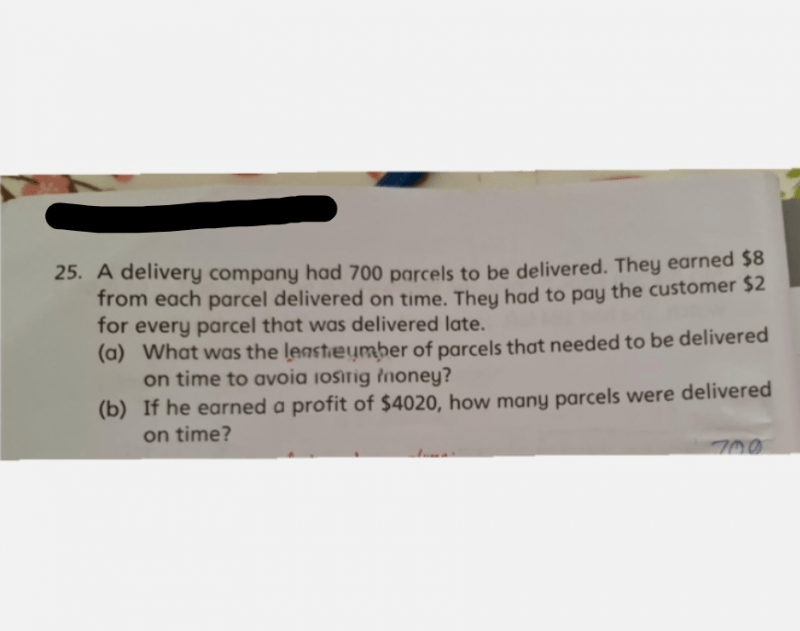# QuestionHi

Can someone help to solve this ? Thanks

There’s multiple methods you can use to solve both parts (a) and (b)

For every parcel delivered on time = + \$8

For every late parcel = – \$2

(a)

Method 1: Algebra

Let the number of parcels delivered on time be x. Thus, the number of late parcels will be (700 – x)

Form an equation with the information provided in the question:

8 (x) + (-2) (700 – x) >= 0 (8 dollars per parcel on time, -2 per late parcel, total sum must be more than or equal to 0 to avoid losing money)

8 x – 1400 + 2 x >= 0

10 x >= 1400

x >= 140

Hence, the least number of parcels which needs to be delivered on time is 140.

Method 2: Ratio?

8 / 2 = 4 (For every 1 parcel delivered on time, 4 parcels can be delivered late without any loss)

5 u = 700

u = 700 / 5

=140

Hence, the least number of parcels which needs to be delivered on time is 140.

In this case, Method 2 seems to be easier, but it’s also because the numbers are “nice” in a sense where 8 is easily divisible by 2. If the numbers get larger or aren’t going to be divisible, Method 1 should be the go-to method, but I’m not sure if P5s would have learnt Algebra.

(b)

Method 1: Guess-and-Check

This should be the method which most schools teach. Find a random number and start working from there. In this case, parcels on time should be >500, since 500 x 8  is 4000, which is less than the profit in the question, so we can start with a number like 550. Subsequently, compare the total profits and adjust the numbers accordingly.

 Parcels on time Profit Late Parcels Loss Total Profit Check 550 4400 150 -300 4100 > 4020 x 540 4320 160 -320 4000 < 4020 x 541 4328 159 -318 4010 < 4020 x 542 4336 158 -316 4020 /

By guess-and-check, 542 parcels were delivered on time.

Method 2: Big-brained method

This method was taught to me when I was in Primary School and I loved it, and never had to resort to guessing-and-checking, which would sometimes be time-consuming. This method requires a bit of understanding to work, so if you can’t grasp it then you can just rely on guess-and-check.

If you take a look at the last three rows of our guess-and-check, you can see that by adding one parcel on time and removing on late parcel, we’re shifting the total profit by increments of 10. This number actually comes from 8 – (-2) which is the profit difference between a parcel on time and a late parcel.

Keeping this in mind, we can start by assuming that all the parcels were delivered on time.

Total profit would be 8 x 700 = 5600.

Now, we know that the actual profit isn’t 5600, because for every late parcel, we have to deduct \$10 from this total. (You lose the \$8 you would’ve gotten, plus you incur a \$2 penalty).

By using 5600-4020, we can see how much we’re deducting from the total.

5600-4020 = 1580

1580 / 10 = 158 (This means that we’re taking away \$10 158 times from the maximum total, which also means there were 158 late parcels)

Hence, total parcels delivered on time = 700 – 158 = 542//

These type of questions are really popular; sometimes they might say there’s a total of x legs on a farm which has cows and chickens, then you need to find out how many of each type there are etc. so if you can understand Method 2 well and practice it proficiently, then it’s an advantage you will have in your exams, because it’s less time-consuming than trying out various numbers.